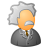# lat long and values of each pixel

1356
3
11-24-2013 06:28 AMNew Contributor
Hi

Is there any way to get the x, y coordinates of each pixel and the corresponding pixel value in arcmap 10 (and whether I can export to excel)

Thanks a ot
Tags (3)
3 RepliesOccasional Contributor III
It can be done using Python. Take a look at gis stackexchange forum post.byMVP Frequent Contributor
Yes,
RasterToPoint & Add_XY are probably what you need. But if the Raster is large then that is a lot of points.
Why would you need to look at it in Excel anyway?

NNew Contributor
Yes,
RasterToPoint & Add_XY are probably what you need. But if the Raster is large then that is a lot of points.
Why would you need to look at it in Excel anyway?

N

Well I am trying to calculate  evapotranspiration for each grid for the month of January using the formula:

PE = 16*C*(10Tm/I)^a

where PE= Potential evapotranspiration
Tm= January average temperature
I= Σ (Tm / 5)^1.51
a= (67.5 x 10-8 * I^3) �?? (77.1 x 10-6 * I^2) + (0.0179 * I) + 0.492
C= daylight coefficient
the value of C is calculated as:

C = 0.0721x + 0.5414 where x is the lat value of each grid

So I wanted to have lat value of each grid, use it to calculate C for each grid and use the C for calculating PE for the grid. It sounds way to complicated. Any idea if this can be done using raster calculator?